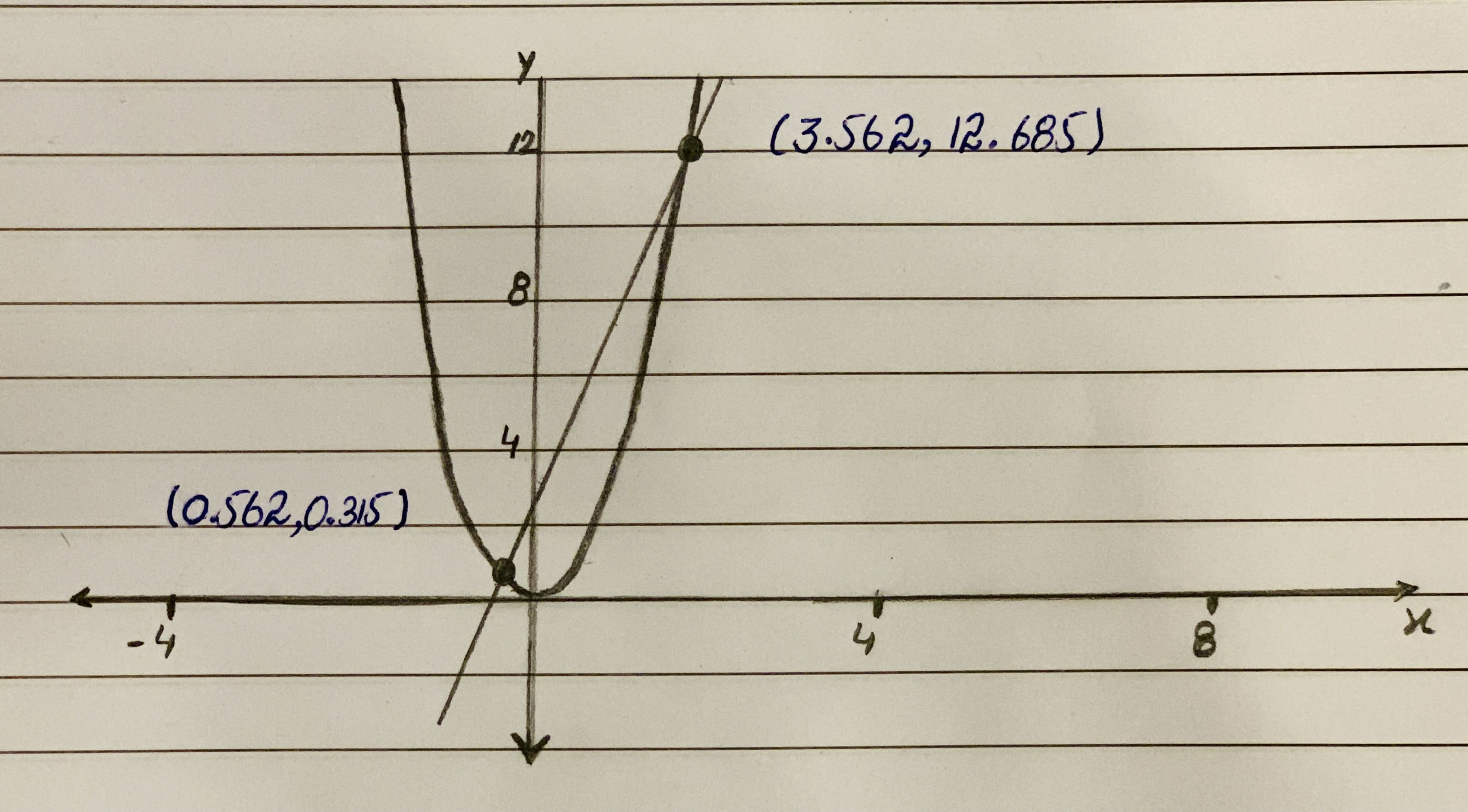# Solve the following linear and quadratic systems of equations:y + 3x = -2 or 3x +2 = yy = x^2a. Show all work in solving your system of equations algebraically.b. Graph your system of equations and show the solution graphically to verify your solution.Tazmin Horton 2020-10-19 Answered

Solve the following linear and quadratic systems of equations:

$y={x}^{2}$
a. Show all work in solving your system of equations algebraically.
b. Graph your system of equations and show the solution graphically to verify your solution.

You can still ask an expert for help

• Live experts 24/7
• Questions are typically answered in as fast as 30 minutes
• Personalized clear answers

Solve your problem for the price of one coffee

• Math expert for every subject
• Pay only if we can solve itliingliing8

Given Data:
Linear equation: $y=3x+2$ ……(1)
Quadratic equation: $y=x2$ ……(2)
(a)
Substitute x2 for y in the linear equation and solve
$y=3x+2$
${x}^{2}=3x+2$
${x}^{2}-3x-2=0$
$x=\left(-\left(-3\right)±\sqrt{\left(-3{\right)}^{2}-4\left(1\right)\left(-2\right)\right)/\left(2\left(1\right)}$
$x=3±\sqrt{17}/2$
$x=3.562$ and $x=0.562$
substitute 3.562 for x in equation 2
$y={x}^{2}$
$y=\left(3.562{\right)}^{2}$
$y=12.685$substitute -0.562 for x in equation 2
$y={x}^{2}$
$y=\left(-0.562{\right)}^{2}$
$y=0.315$
From above, the intersection points of line and curve are (-0.562, 0.315) and (3.562, 12.685).
(b)
The graph of line and curve is shown in the figure below.